# MCQ in Control Systems Part 1 | Engineering Board Exam

(Last Updated On: August 6, 2022)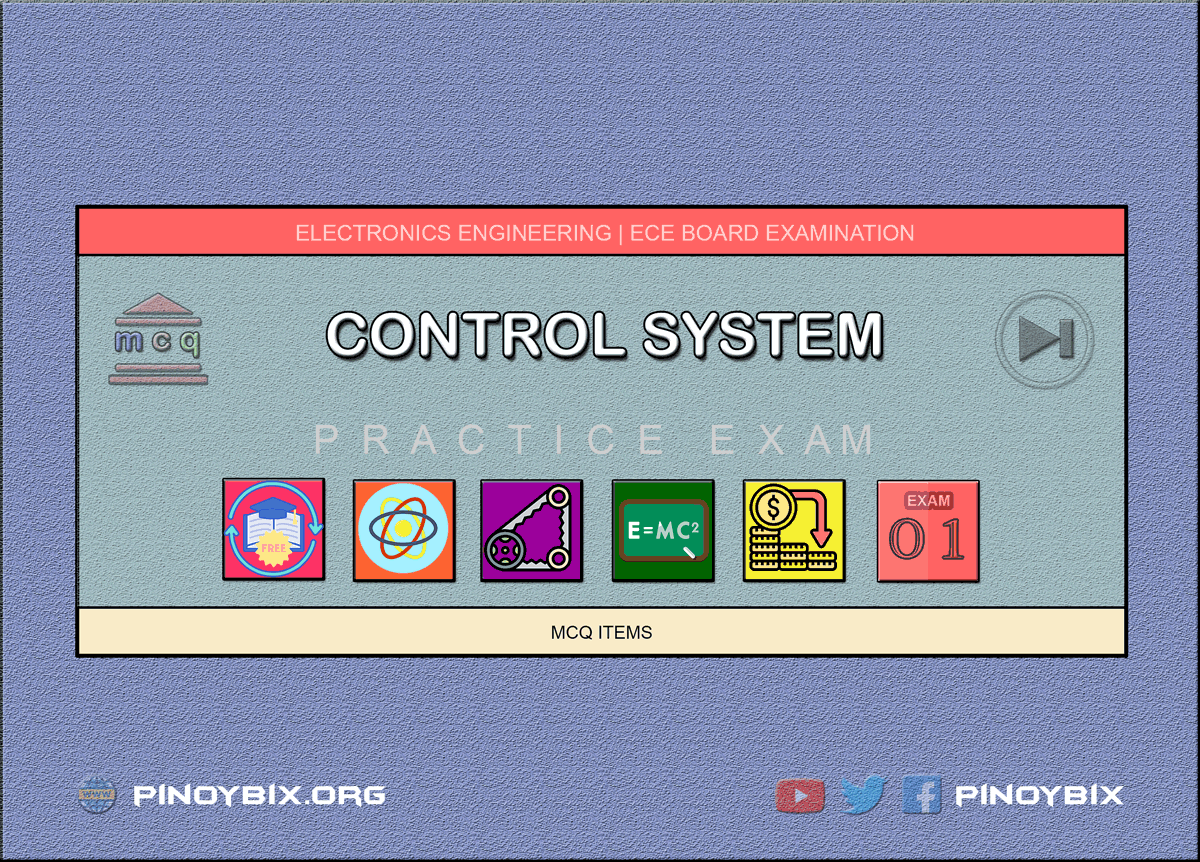This is the Multiple Choice Questions Part 1 of the Series in Control Systems as one of the Electronics Engineering topic. In Preparation for the ECE Board Exam make sure to expose yourself and familiarize in each and every questions compiled here taken from various sources including but not limited to past Board Exam Questions in Electronics Engineering field, Electronics Books, Journals and other Electronics References.

#### Start Practice Exam Test Questions Part 1 of the Series

Choose the letter of the best answer in each questions.

1. What is the algebraic sum of the reference input and feedback?

A. error signal

B. error detector

C. controlled system

D. controlled output

Solution:

2. Feedback control systems are referred to as closed loop systems.

A. true

B. false

Solution:

3. Which principle does the linear system follow?

A. principle of energy conservation

B. principle of mass conservation

C. principle of electromagnetism

D. principle of superposition

Solution:

4. ______ control systems have unpredictable & non-repeatable.

A. static

B. dynamic

C. deterministic

D. stochastic

Solution:

5. The pressure inside the furnace is measured by

A. gauge

B. thermometer

C. manometer

D. barometer

Solution:

6. On what difference does the pneumatic system works?

A. speed

B. pressure

C. area

D. length

Solution:

7. In a thermal system, the temperature of the medium is

A. increasing

B. decreasing

C. zero

D. uniform

Solution:

8. How many parameters does process control refer to?

A. 1

B. 3

C. 5

D. 7

Solution:

9. What is the effect of feedback in the overall gain of the system?

A. increases

B. decreases

C. zero

D. no change

Solution:

10. In a temperature control system, what conversion in signal takes place?

A. digital to analog

B. analog to digital

C. error to digital

D. error to analog

Solution:

11. Which of the following are the not characteristics of the closed loop systems?

A. it does not compensate for disturbance

B. it reduces the sensitivity of plant-parameter variations

C. it does not involve output measurements

D. it does not has the ability to control the system transient response

Solution:

12. Which one of the following effects in the system is not caused by negative feedback?

A. reduction in gain

B. increased in bandwidth

C. increase in distortion

D. reduction in output impedance

Solution:

13. Which of the statement is correct with regard to the bandwidth of the control loop system:

A. in systems where the low frequency magnitude in 0 dB on the bode diagram, the bandwidth is measured at the -3 dB frequency

B. the bandwidth is the measurement of the accuracy of the closed loop system

C. the stability is proportional to the bandwidth

D. the system with larger bandwidth provides slower step response and lower fidelity ramp response

Solution:

14. Which of the statements are the advantages of closed loop systems over the open loop system:

A. the overall reliability of the open loop system is more than the closed loop system is more than closed loop system.

B. the transient response in closed loop system decays more quickly than in open loop system.

C. in open loop system closing the loop increases the gain of the system

D. in open loop system the effect of parameter variation is reduced

Solution:

15. In control system excessive bandwidth is not employed because

A. noise is proportional to bandwidth

B. it leads to low relative stability

C. it leads to slower time response

D. noise is proportional to the square of the bandwidth

Solution:

16. Feedback control system is basically

A. high pass filter

B. low pass filter

C. band pass filter

D. band stop filter

Solution:

17. Which of the following will not decrease as a result of introduction of negative feedback?

A. instability

B. bandwidth

C. overall gain

D. distortion

Solution:

18. As compared to the closed loop system, an open loop system is

A. more stable but less accurate

B. less stable as well as less accurate

C. more stable as well as more accurate

D. less stable but more accurate

Solution:

19. Insertion of negative feedback in control system affects:

A. the transient response to vanish uniformly

B. the transient response to decay very fast

C. no change in transient response

D. the transient response decays at slow rate

Solution:

20. Open loop system is ______ stable than closed loop system

A. more

B. less

C. inclined

D. exponential

Solution:

21. For the gain feedback system, does not affect the system output if KG is :

A. small

B. negative

C. one

D. very large

Solution:

22. Determine the sensitivity of the overall transfer function for the system shown in the figure below, at w=1 rad/sec with respect to the feedback path transfer function.

A. 1.11

B. -1.11

C. 2.22

D. -2.22

Solution:

23. Primary purpose of using Feedback is :

A. to reduce the sensitivity of the system to parameter variations.

B. to increase the bandwidth of the system

C. to reduce the noise and distortion of the system

D. to increase stability of the system

Solution:

24. Which of the following is not the feature of modern control system?

A. quick response

B. accuracy

C. correct power level

D. no oscillation

Solution:

25. The output of the feedback control system must be a function of:

A. reference input

B. reference output

C. output and feedback signal

D. input and feedback signal

Solution:

26. The principle of homogeneity and superposition are applied to:

A. linear time invariant systems

B. nonlinear time invariant systems

C. linear time variant systems

D. nonlinear time invariant systems

Solution:

27. In continuous data systems:

A. data may be continuous function of time at all points in the system

B. data is necessarily a continuous function of time at all points in the system

C. data is continuous at the inputs and output parts of the system but not necessarily during intermediate processing of the data

D. only the reference signal is continuous function of time

Solution:

28. A linear system at rest is subject to an input signal r(t)=1-e-t. The response of the system for t>0 is given by c(t)=1-e-2t. The transfer function of the system is:

A. (s+2)/(s+1)

B. (s+1)/(s+2)

C. 2(s+1)/(s+2)

D. (s+1)/2(s+2)

Solution:

29. A transfer function has two zeroes at infinity. Then the relation between the numerator (N) and the denominator degree (M) of the transfer function is:

A. n=m+2

B. n=m-2

C. n=m+1

D. n=m-1

Solution:

30. When deriving the transfer function of a linear element

A. both initial conditions and loading are taken into account

B. initial conditions are taken into account, but the element is assumed to be not loaded

C. initial conditions are assumed to be zero but loading is taken into account

D. initial conditions are assumed to be zero and the element is assumed to be not loaded

Solution:

31. If the initial conditions for a system are inherently zero, what does it physically mean?

A. the system is at rest but stores energy

B. the system is working but does not store energy

C. the system is at rest or no energy is stored in any of its part

D. the system is working with zero reference input

Solution:

32. A signal flow graph is the graphical representation of the relationships between the variables of set linear algebraic equations.

A. true

B. false

Solution:

33. Use mason’s gain formula to find the transfer function of the given signal flow graph: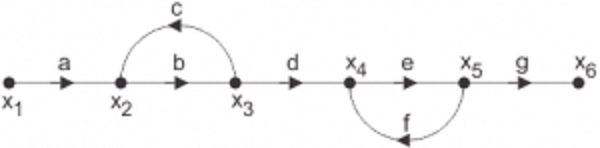A. abd/1-(ac)

B. abdeg/1-(bc+ef)+bcef

C. abd/1-(bc+ef)+bcef

Solution:

34. Use mason’s gain formula to find the transfer function of the following signal flow graph: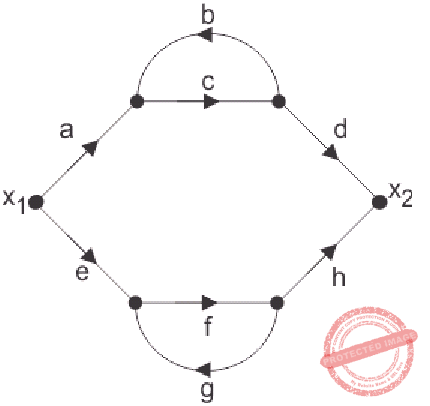A. abcd+efg/1-cd-fg-cdfg

B. acdfg+bcefg/1-cd-fg-cdfg

C. abef+bcd/1-cd-fg-cdfg

Solution:

35. Loop which do not possess any common node are said to be ______ loops.

A. forward gain

B. touching loops

C. non touching loops

D. feedback gain

Solution:

36. Signal flow graphs:

A. they apply to linear systems

B. the equation obtained may or may not be in the form of cause or effect

C. arrows are not important in the graph

D. they cannot be converted back to block diagram

Solution:

37. Signal flow graphs are reliable to find transfer function than block diagram reduction technique.

A. true

B. false

Solution:

38. The relationship between an input and output variable of a signal flow graph is given by the net gain between the input and output node is known as the overall

A. overall gain of the system

B. stability

C. bandwidth

D. speed

Solution:

39. Use mason’s gain formula to calculate the transfer function of given figure: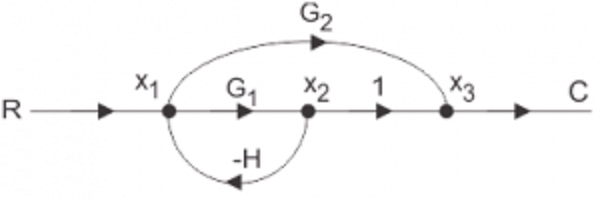A. g1/1+g2h

B. g1+g2/1+g1h

C. g2/1+g1h

D. none of the mentioned

Solution:

40. Use mason’s gain formula to find the transfer function of the given figure: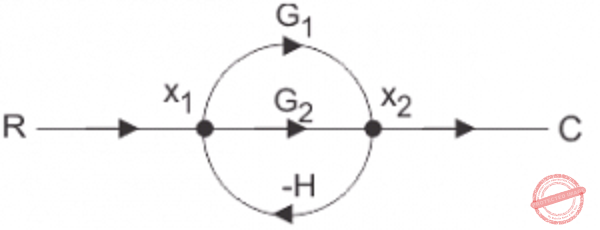A. g1+g2

B. g1+g1/1-g1h+g2h

C. g1+g2/1+g1h+g2h

D. g1-g2

Solution:

41. Regenerative feedback implies feedback with

A. oscillations

B. step input

C. negative sign

D. positive sign

Solution:

explanation:-regenerative feedback that is the positive feedback implies feedback with positive sign and for complementary root locus is for the regenerative feedback.

42. The output of a feedback control system must be a function of

A. reference and output

B. reference and input

C. input and feedback signal

D. output and feedback signal

Solution:

43. A control system with excessive noise, is likely to suffer from

A. saturation in amplifying stages

B. loss of gain

C. vibrations

D. oscillations

Solution:

44. Zero initial condition for a system means

A. input reference signal is zero

B. zero stored energy

C. initial movement of moving parts

D. system is at rest and no energy is stored in any of its components

Solution:

45. Transfer function of a system is used to calculate which of the following?

A. the order of the system

B. the time constant

C. the output for any given input

D. the steady state gain

Solution:

46. The band width, in a feedback amplifier.

A. remains unaffected

B. decreases by the same amount as the gain increase

C. increases by the same amount as the gain decrease

D. decreases by the same amount as the gain decrease

Solution:

47. On which of the following factors does the sensitivity of a closed loop system to gain changes and load disturbances depend?

A. frequency

B. loop gain

C. forward gain

D. all of the mentioned

Solution:

48. The transient response, with feedback system,

A. rises slowly

B. rises quickly

C. decays slowly

D. decays quickly

Solution:

49. The second derivative input signals modify which of the following?

A. the time constant of the system

B. damping of the system

C. the gain of the system

D. the time constant and suppress the oscillations

Solution:

50. The transient response, yt(t), of a system becomes ______ as t tends to infinity.

A. 0

B. 1

C. infinity

D. undefined

Solution:

### Complete List of MCQ in Electronics Engineering per topic

PinoyBIX Engineering. © 2014-2021 All Rights Reserved | How to Donate? |